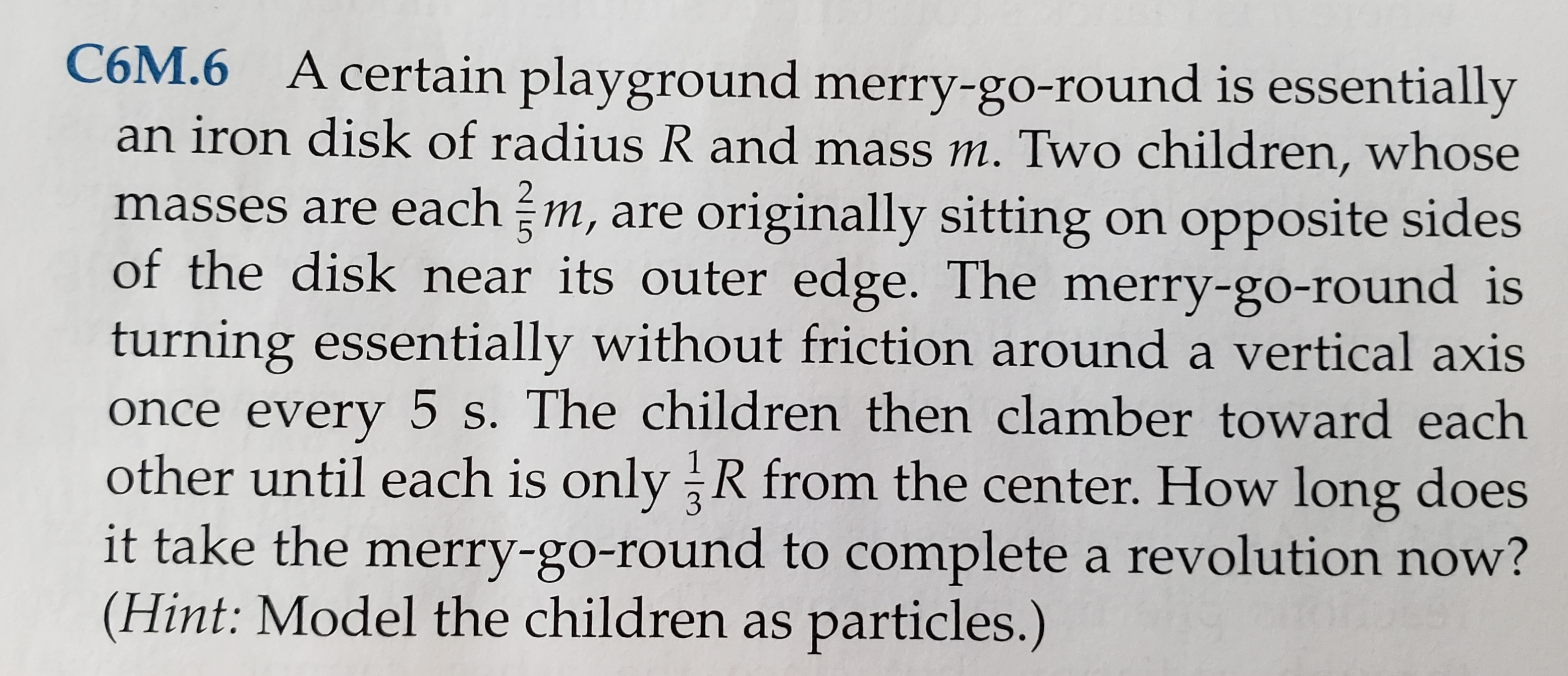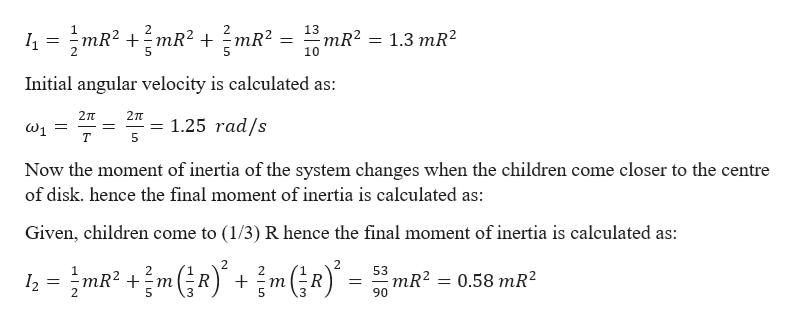# C6M.6 A certain playground merry-go-round is essentiallyan iron disk of radius R and mass m. Two children, whosemasses are each m, are originally sitting on opposite sidesof the disk near its outer edge. The merry-go-round isturning essentially without friction around a vertical axisonce every 5 s. The children then clamber toward eachother until each is only R from the center. How long doesit take the merry-go-round to complete a revolution now?(Hint: Model the children as particles.)

Question
3 viewshelp_outlineImage TranscriptioncloseC6M.6 A certain playground merry-go-round is essentially an iron disk of radius R and mass m. Two children, whose masses are each m, are originally sitting on opposite sides of the disk near its outer edge. The merry-go-round is turning essentially without friction around a vertical axis once every 5 s. The children then clamber toward each other until each is only R from the center. How long does it take the merry-go-round to complete a revolution now? (Hint: Model the children as particles.) fullscreen
check_circle

Step 1

Given information:

Radius of the iron disk = R

Mass of the disk = m

Distance of each child from the centre initially = R

Mass of each child = (2/5) m

Initial time taken by the disk to make one rotation (T) = 5 s

Let the initial, moment of inertia and angular velocity = I1, ω1

Let the final, moment of inertia and angular velocity = I2, ω2

Step 2

Hence the initial moment of inertia is given by:help_outlineImage Transcriptionclose2 2 mR2 13 mR2 10 1.3 mR2 Initial angular velocity is calculated as: 2TT 2TT 1.25 rad/s T Now the moment of inertia of the system changes when the children come closer to the centre of disk. hence the final moment of inertia is calculated as: Given, children come to (1/3) R hence the final moment of inertia is calculated as: 2 2 53 mR2 m mR2 90 0.58 mR2 12 R 2 3 fullscreen
Step 3

Now, according to law of conservation of angular momen...

### Want to see the full answer?

See Solution

#### Want to see this answer and more?

Solutions are written by subject experts who are available 24/7. Questions are typically answered within 1 hour.*

See Solution
*Response times may vary by subject and question.
Tagged in

### Angular Motion